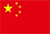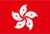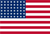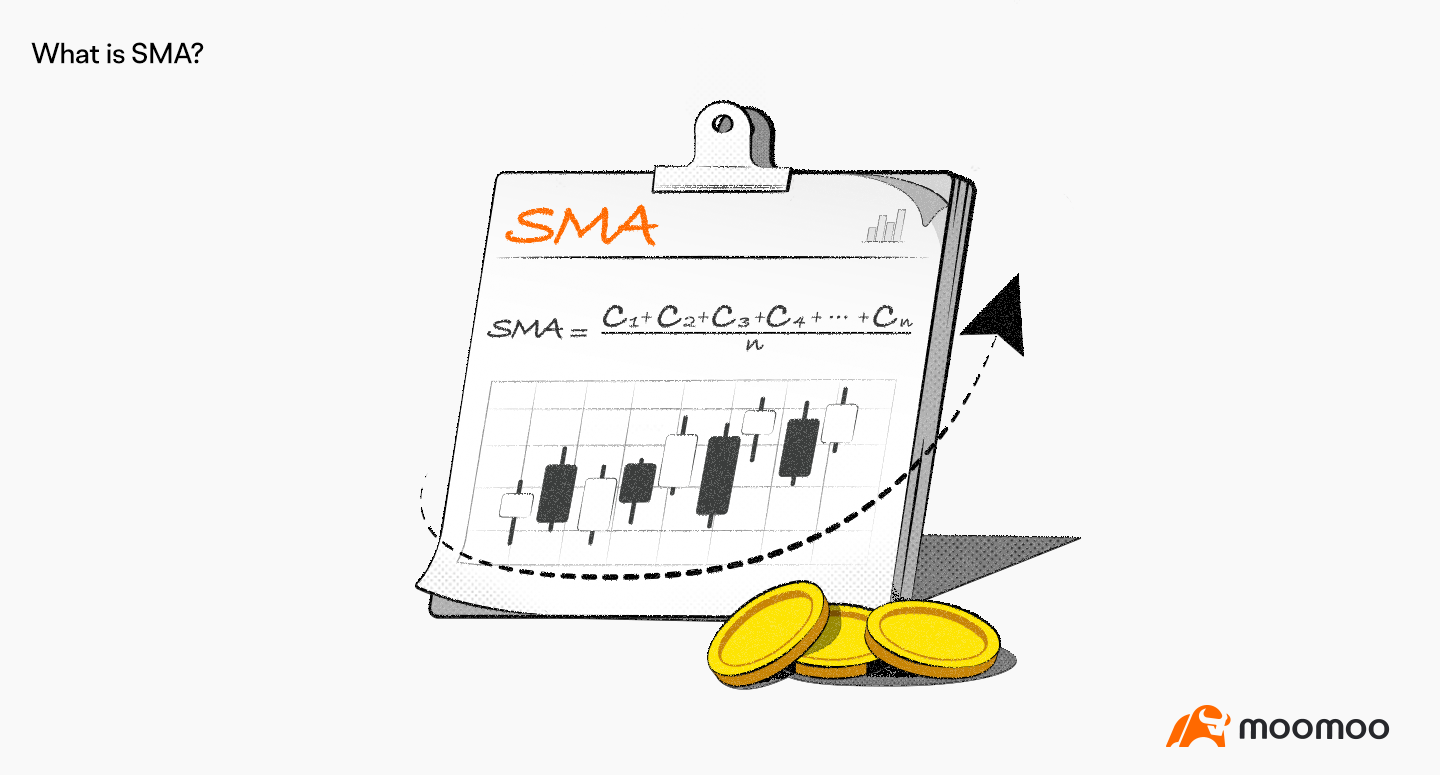Scanning QR Code+
Congratulations
You've successfully got advanced quotes worth 5000 HKD
• Home
• Quotes
• News
• Community
• Learn
• Help Center
• Language
•••EN

# What Is Simple Moving Average (SMA)?

Views 52692022.09.29# Core points

The ● simple moving average is one of the most basic indicators for observing price changes.

● when the short-term average is above the longer-term average, indicating that the short-term price is stronger, the strategy can tend to be long.

If ● encounters extreme fluctuations in the short term, the simple moving average may appear obvious distortion.

# Concept understanding

Simple moving average (Simple Moving Average), also known as "arithmetic moving average", refers to the numerical connection calculated by the simple average of the price in a specific period of time, which is generally calculated by the closing price of the stock. The simple moving average is one of the most basic indicators for observing price fluctuations.

# The calculation Formula of simple moving average

Take the 5-day simple average as an example, first determine a continuous 5-day cycle, simply add up the daily closing price, and then divide by 5 to get the starting position of the simple moving average.

Then, for each day that continues backward, the closing price of the first day is removed, the closing price of the sixth day is increased, and the second price position is calculated by simply adding and dividing by 5. And so on, all the values are finally connected to form a simple moving average.

# How to use a simple moving average

When conducting primary technical analysis, most investors will choose to use the simple moving average to set up a basic trading system and use the shorter-term average in the system as the buying and selling signal.

For example, when the short-term average (5th line) crosses the longer-term average (30th, 60th day), indicating that the short-term price is stronger, the strategy may tend to be long.

On the contrary, when the short-term average (5th line) falls below the longer-term average (30th, 60th day), indicating that the short-term price is weaker, the strategy can tend to be short.

It is worth noting that the simple moving average gives the same time weight to the daily closing price, without taking into account the difference between recent and forward share prices. If you encounter short-term extreme fluctuations, the index may be significantly distorted.

Disclaimer: The above content does not constitute any act of financial product marketing, investment offer, or financial advice. Before making any investment decision, investors should consider the risk factors related to investment products based on their own circumstances and consult professional investment advisors where necessary.